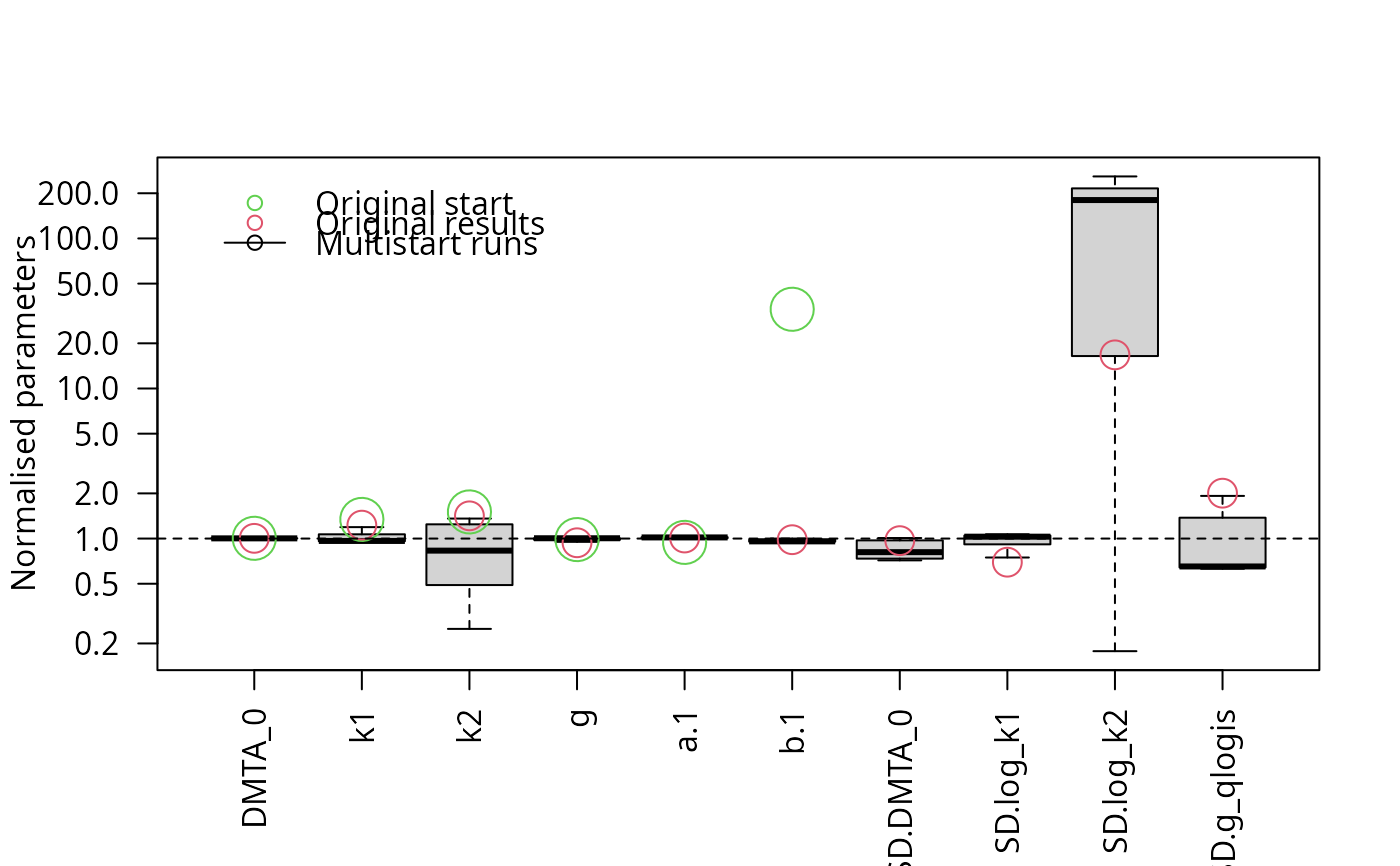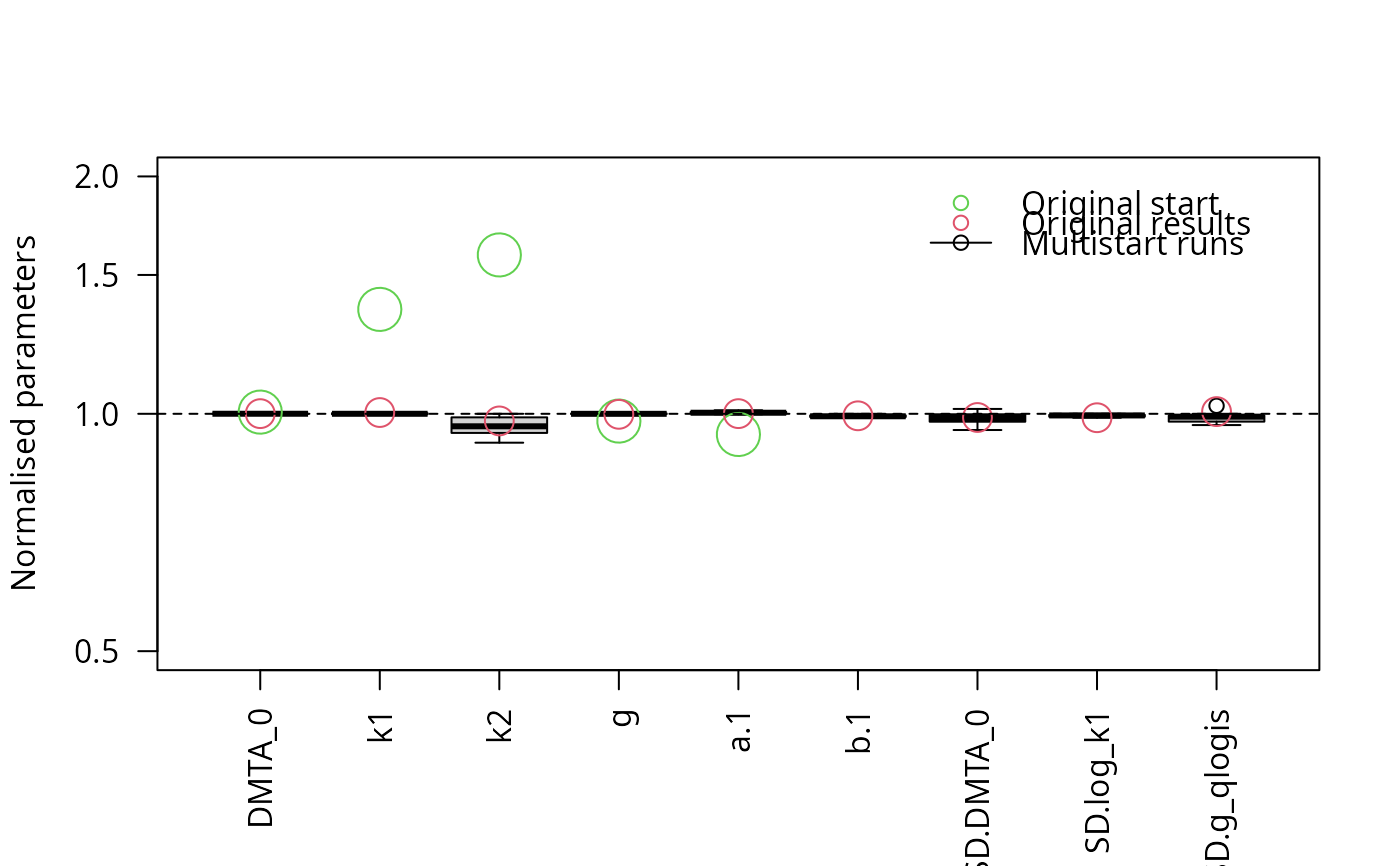The purpose of this method is to check if a certain algorithm for fitting nonlinear hierarchical models (also known as nonlinear mixed-effects models) will reliably yield results that are sufficiently similar to each other, if started with a certain range of reasonable starting parameters. It is inspired by the article on practical identifiabiliy in the frame of nonlinear mixed-effects models by Duchesne et al (2021).

multistart(
object,
n = 50,
cores = if (Sys.info()["sysname"] == "Windows") 1 else parallel::detectCores(),
cluster = NULL,
...
)

# S3 method for saem.mmkin
multistart(object, n = 50, cores = 1, cluster = NULL, ...)

# S3 method for multistart
print(x, ...)

best(object, ...)

# S3 method for default
best(object, ...)

which.best(object, ...)

# S3 method for default
which.best(object, ...)

## Arguments

object

The fit object to work with

n

How many different combinations of starting parameters should be used?

cores

How many fits should be run in parallel (only on posix platforms)?

cluster

A cluster as returned by parallel::makeCluster to be used for parallel execution.

...

Passed to the update function.

x

The multistart object to print

## Value

A list of saem.mmkin objects, with class attributes 'multistart.saem.mmkin' and 'multistart'.

The object with the highest likelihood

The index of the object with the highest likelihood

## Examples

# \dontrun{
library(mkin)
dmta_ds <- lapply(1:7, function(i) {
ds_i <- dimethenamid_2018$ds[[i]]$data
ds_i[ds_i$name == "DMTAP", "name"] <- "DMTA" ds_i$time <- ds_i$time * dimethenamid_2018$f_time_norm[i]
ds_i
})
names(dmta_ds) <- sapply(dimethenamid_2018$ds, function(ds) ds$title)
dmta_ds[["Elliot"]] <- rbind(dmta_ds[["Elliot 1"]], dmta_ds[["Elliot 2"]])
dmta_ds[["Elliot 1"]] <- dmta_ds[["Elliot 2"]] <- NULL

f_mmkin <- mmkin("DFOP", dmta_ds, error_model = "tc", cores = 7, quiet = TRUE)
f_saem_full <- saem(f_mmkin)
f_saem_full_multi <- multistart(f_saem_full, n = 16, cores = 16)
parplot(f_saem_full_multi, lpos = "topleft")illparms(f_saem_full)
#>  "sd(log_k2)"

f_saem_reduced <- update(f_saem_full, no_random_effect = "log_k2")
illparms(f_saem_reduced)
# On Windows, we need to create a cluster first. When working with
# such a cluster, we need to export the mmkin object to the cluster
# nodes, as it is referred to when updating the saem object on the nodes.
library(parallel)
cl <- makePSOCKcluster(12)
f_saem_reduced_multi <- multistart(f_saem_reduced, n = 16, cluster = cl)
parplot(f_saem_reduced_multi, lpos = "topright")stopCluster(cl)
# }